## Digital-Logic-Design

 Question 1

In 16-bit 2’s complement representation, the decimal number -28 is:

 A 1111 1111 1110 0100 B 1111 1111 0001 1100 C 0000 0000 1110 0100 D 1000 0000 1110 0100
Digital-Logic-Design       Number-Systems       GATE 2019       Video-Explanation
Question 1 Explanation:
+28 = 0000 0000 0001 1100

1’s complement = 1111 1111 1110 0011
2’s complement = 1’s complement + 1

2’s complement = 1111 1111 1110 0100 = (-28)
 Question 2

Two numbers are chosen independently and uniformly at random from the set {1, 2, …, 13}. The probability (rounded off to 3 decimal places) that their 4-bit (unsigned) binary representations have the same most significant bit is ______.

 A 0.502 B 0.461 C 0.402 D 0.561
Digital-Logic-Design       Number-Systems       GATE 2019       Video-Explanation
Question 2 Explanation:
1 – 0001
2 – 0010
3 – 0011
4 – 0100
5 – 0101
6 – 0110
7 – 0111
8 – 1000
9 – 1001
10 – 1010
11 – 1011
12 – 1100
13 – 1101
The probability that their 4-bit binary representations have the same most significant bit is
= P(MSB is 0) + P(MSB is 1)
= (7×7)/(13×13) + (6×6)/(13×13)
= (49+36)/169
= 85/169
= 0.502
 Question 3

Consider Z = X – Y, where X, Y and Z are all in sign-magnitude form. X and Y are each represented in n bits. To avoid overflow, the representation of Z would require a minimum of:

 A n bits B n + 2 bits C n – 1 bits D n + 1 bits
Digital-Logic-Design       Number-Systems       GATE 2019       Video-Explanation
Question 3 Explanation:
In case of addition of two numbers with the same sign, there is a chance of overflow.
To store overflow/carry bit there should be extra space to accommodate it.
Hence, Z should be n+1 bits.
 Question 4

Which one of the following is NOT a valid identity?

 A (x + y) ⊕ z = x ⊕ (y + z) B (x ⊕ y) ⊕ z = x ⊕ (y ⊕ z) C x ⊕ y = x + y, if xy = 0 D x ⊕ y = (xy + x’y’)’
Digital-Logic-Design       Boolean-Expression       GATE 2019       Video-Explanation
Question 4 Explanation:
Let x=1, y=1, z=0.
(x+y) ⊕ z = (1+1)⊕ 0 = 1 ⊕ 0 = 1
x ⊕ (y+z) = 1⊕(1+0) = 1 ⊕ 1 = 0
So,
(x+y) ⊕ z ≠ x ⊕ (y+z)
 Question 5

What is the minimum number of 2-input NOR gates required to implement a 4-variable function function expressed in sum-of-minterms form as f = Σ(0, 2, 5, 7, 8, 10, 13, 15)? Assume that all the inputs and their complements are available.

 A 2 B 4 C 7 D 1 E 3(Option not given)
Digital-Logic-Design       Logic-Gates       GATE 2019       Video-Explanation
Question 5 Explanation:
f = Σ(0, 2, 5, 7, 8, 10, 13, 15)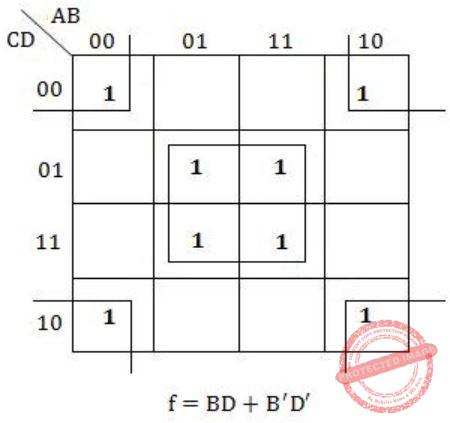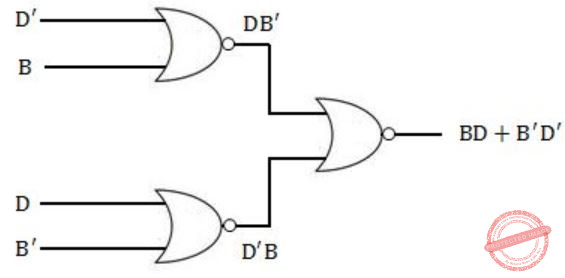Question 6

Consider three 4-variable functions f1, f2 and f3, which are expressed in sum-of-minterms as

```f1 = Σ(0, 2, 5, 8, 14),  f2 = Σ(2, 3, 6, 8, 14, 15),  f3 = Σ(2, 7, 11, 14)
```

For the following circuit with one AND gate and one XOR gate, the output function f can be expressed as: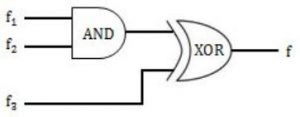A Σ (2, 14) B Σ (7, 8, 11) C Σ (2, 7, 8, 11, 14) D Σ (0, 2, 3, 5, 6, 7, 8, 11, 14, 15)
Digital-Logic-Design       Logic-Gates       GATE 2019       Video-Explanation
Question 6 Explanation:
f1*f2 = ∑(2,8,14)
f3 = ∑(2,7,11,14)
f1*f2 ⊕ f3 = ∑(2,8,14) ⊕ ∑(2,7,11,14)
= ∑(8,7,11)
(Note: Choose the terms which are not common)
 Question 7

Let ⊕ and ⊙ denote the Exclusive OR and Exclusive NOR operations, respectively. Which one of the following is NOT CORRECT?

 ABCDDigital-Logic-Design       Logic-Gates       GATE 2018       Video-Explanation
Question 7 Explanation:Question 8

Consider the sequential circuit shown in the figure, where both flip-flops used are positive edge-triggered D flip-flops.The number of states in the state transition diagram of this circuit that have a transition back to the same state on some value of “in” is ______

 A 2 B 3 C 4 D 5
Digital-Logic-Design       Sequential-Circuits       GATE 2018       Video-Explanation
Question 8 Explanation:
Let,Now lets draw characteristic table,
D1 = Q0
D0 = inQuestion 9
Consider the unsigned 8-bit fixed point binary number representation below,
b7 b6 b5 b4 b3 b2 b1 b0
where the position of the binary point is between b3 and b2 . Assume b7 is the most significant bit.
Some of the decimal numbers listed below cannot be represented exactly in the above representation:
(i) 31.500
(ii) 0.875
(iii) 12.100
(iv) 3.001
Which one of the following statements is true?
 A None of (i), (ii), (iii), (iv) can be exactly represented B Only (ii) cannot be exactly represented C Only (iii) and (iv) cannot be exactly represented D Only (i) and (ii) cannot be exactly represented
Digital-Logic-Design       Number-Systems       GATE 2018       Video-Explanation
Question 9 Explanation:
(i) (31.5)10 = (11111.100)2 = 24 + 23 + 22 + 21 + 20 + 2-1
= 16 + 8 + 4 + 2 + 1 + 0.5
= (31.5)10
(ii) (0.875)10 = (00000.111)2
= 2-1 + 2-2 + 2-3
= 0.5 + 0.25 + 0.125
= (0.875)10
(iii) (12.100)10
It is not possible to represent (12.100)10
(iv) (3.001)10 It is not possible to represent (3.001)10
 Question 10

Consider the minterm list form of a Boolean function F given below.

F(P, Q, R, S) = Σm(0, 2, 5, 7, 9, 11) + d(3, 8, 10, 12, 14)

Here, m denotes a minterm and d denotes a don’t care term. The number of essential prime implicants of the function F is _______.

 A 3 B 4 C 5
Digital-Logic-Design       K-Map       GATE 2018       Video-Explanation
Question 10 Explanation:
f = Σ(0, 2, 5, 7, 9, 11) + d(3, 8, 10, 12, 14)There are 3 prime implicant i.e., P’QS, Q’S’ and PQ’ and all are essential.
Because 0 and 2 are correct by only Q’S’, 5 and 7 are covered by only P’QS and 8 and 9 are covered by only PQ’.
 Question 11

The n-bit fixed-point representation of an unsigned real number X uses f bits for the fraction part. Let i = n-f. The range of decimal values for X in this representation is

 A 2-f to 2i B 2-f to (2i – 2-f) C 0 to 2i D 0 to (2i – 2-f )
Digital-Logic-Design       Number-Systems       GATE 2017 [Set-1]       Video-Explanation
Question 11 Explanation:
Size of the fixed point number → n-bits
Number of bits in fraction part → f-bits
Number of bits in integer part → (n – f) bits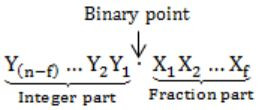Minimum value:
000…0.000…0 = 0
Maximum value:= (2 n-f – 1) + (1 – 2 -f
= (2n-f – 2 -f)
= (2i – 2 -f )
 Question 12

When two 8-bit numbers A7…A0 and B7…B0 in 2’s complement representation (with A0 and B0 as the least significant bits) are added using a ripple-carry adder, the sum bits obtained are S7…S0 and the carry bits are C7…C0. An overflow is said to have occurred if

 A the carry bit C7 is 1 B all the carry bits (C7,…,C0) are 1 CDDigital-Logic-Design       Ripple-Carry-Adder       GATE 2017 [Set-1]       Video-Explanation
Question 12 Explanation:
⇾ Overflow may occur when numbers of same sign are added
i.e., A7 = B7
⇾ Overflow can be detected by checking carry into the sign bits (Cin) and carry out of the sign bits (Cout).
⇾ Overflow occurs iff A7 = B7 and Cin ≠ Cout
These conditions are equivalent toConsider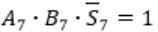Here A7 = B7 = 1 and S7 = 0
This happens only if Cin = 0Carry out Cout=1 when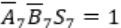Similarly, in case ofCin=1 and Cout will be 0.Question 13

Consider the Karnaugh map given below, where X represents “don’t care” and blank represents 0.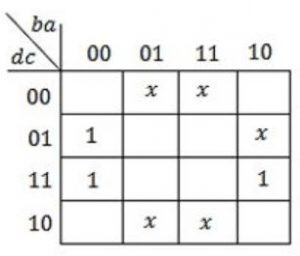Assume for all inputs, the respective complements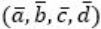are also available. The above logic is implemented using 2-input NOR gates only. The minimum number of gates required is _________.

 A 1 B 2 C 3 D 4
Digital-Logic-Design       K-Map       GATE 2017 [Set-1]       Video-Explanation
Question 13 Explanation:
Given K-Map represents the function f(a, b, c, d) = a’c = a'(c’)’ = (a + c’)’
As all variables and their complements are available we can implement the function with only one NOR Gate.
 Question 14

Consider a combination of T and D flip-flops connected as shown below. The output of the D flip-flop is connected to the input of the T flip-flop and the output of the T flip-flop is connected to the input of the D flip-flop.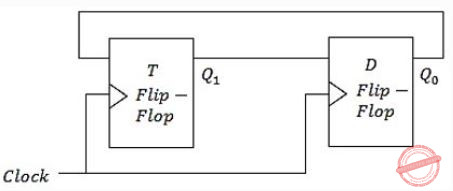Initially, both Q0 and Q1 are set to 1 (before the 1st clock cycle). The outputs

 A Q1Q0 after the 3rd cycle are 11 and after the 4th cycle are 00 respectively B Q1Q0 after the 3rd cycle are 11 and after the 4th cycle are 01 respectively C Q1Q0 after the 3rd cycle are 00 and after the 4th cycle are 11 respectively D Q1Q0 after the 3rd cycle are 01 and after the 4th cycle are 01 respectively
Digital-Logic-Design       Sequential-Circuits       GATE 2017 [Set-1]       Video-Explanation
Question 14 Explanation: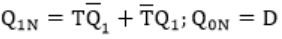Question 15

The representation of the value of a 16-bit unsigned integer X in hexadecimal number system is BCA9. The representation of the value of X in octal number system is

 A 136251 B 736251 C 571247 D 136252
Digital-Logic-Design       Number-Systems       GATE 2017 [Set-2]       Video-Explanation
Question 15 Explanation:
X = (BCA9)16
Each hexadecimal digit is equal to a 4-bit binary number. So convert
X = (BCA9)16 to binaryDivide the binary data into groups 3 bits each because each octal digit is represented by 3-bit binary number.
X = (001 011 110 010 101 001)2
Note: Two zeroes added at host significant position to make number bits of a multiple of 3 (16 + 2 = 18)
X = (136251)8
 Question 16

Given the following binary number in 32-bit (single precision) IEEE-754 format:

00111110011011010000000000000000

The decimal value closest to this floating-point number is

 A 1.45 × 101 B 1.45 × 10-1 C 2.27 × 10-1 D 2.27 × 101
Digital-Logic-Design       Number-Systems       GATE 2017 [Set-2]       Video-Explanation
Question 16 Explanation:For single-precision floating-point representation decimal value is equal to (-1)5 × 1.M × 2(E-127)
S = 0
E = (01111100)2 = (124).
So E – 127 = – 3
1.M = 1.11011010…0
= 20 + 2(-1) + 2(-1) + 2(-4) + 2(-5) + 2(-7)
= 1+0.5+0.25+0.06+0.03+0.007
≈ 1.847
(-1)5 × 1.M × 2(E-127)
= -10 × 1.847 × 2-3
≈ 0.231
≈ 2.3 × 10-1
 Question 17

Consider a quadratic equation x2 – 13x + 36 = 0 with coefficients in a base b. The solutions of this equation in the same base b are x = 5 and x = 6. Then b=________.

 A 8 B 9 C 10 D 11
Digital-Logic-Design       Number-Systems       GATE 2017 [Set-2]       Video-Explanation
Question 17 Explanation:
x2 – 13x + 36 = 0 ⇾(1)
Generally if a, b are roots.
(x – a)(x – b) = 0
x2 – (a + b)x + ab = 0
Given that x=5, x=6 are roots of (1)
So, a + b = 13
ab=36 (with same base ‘b’)
i.e., (5)b + (6)b = (13)b
Convert them into decimal value
5b = 510
610 = 610
13b = b+3
11 = b+3
b = 8
Now check with ab = 36
5b × 6b = 36b
Convert them into decimals
5b × 6b = (b×3) + 610
30 = b × 3 + 6
24 = b × 3
b = 8
∴ The required base = 8
 Question 18

If w, x, y, z are Boolean variables, then which one of the following is INCORRECT?

 A wx + w(x + y) + x(x + y) = x + wy BCD (w + y)(wxy + wyz) = wxy + wyz
Digital-Logic-Design       Boolean-Expressions       GATE 2017 [Set-2]       Video-Explanation
Question 18 Explanation:
Option-A:
wx + w(x + y) + x(x + y)
= (wx + wx) + wy + (x + xy)
= wx + wy + x(1 + y)
= wx + wy + x
= (w + 1)x + wy
= x + wy
Option-B: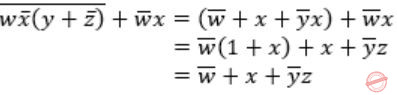Option-C: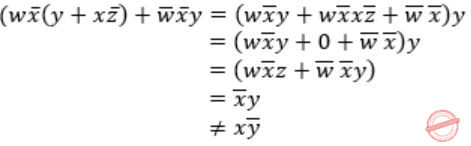Option-D:
(w + y)(wxy + wyz) = wxy + wyz + wxy + wyz = wxy + wyz
 Question 19

Given f(w,x,y,z) = Σm(0,1,2,3,7,8,10) + Σd(5,6,11,15), where d represents the don’t-care condition in Karnaugh maps. Which of the following is a minimum product-of-sums (POS) form of f(w,x,y,z)?

 A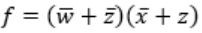BCDDigital-Logic-Design       K-Map       GATE 2017 [Set-2]       Video-Explanation
Question 19 Explanation:
f(w,x,y,z) = Σm(0,1,2,3,7,8,10) + Σd(5,6,11,15)
K-Map for the function f isConsider maxterms in K-map to represent function in product-of-sums (POS) form
f(w,x,y,z) = (w’ + z’)(x’ + z)
 Question 20

Consider a binary code that consists of only four valid code words as given below:

00000, 01011, 10101, 11110

Let the minimum Hamming distance of the code be p and the maximum number of erroneous bits that can be corrected by the code be q. Then the values of p and q are

 A p=3 and q=1 B p=3 and q=2 C p=4 and q=1 D p=4 and q=2
Digital-Logic-Design       Number-Systems       GATE 2017 [Set-2]       Video-Explanation
Question 20 Explanation:
Hamming distance of a code is minimum distance between any two code words.
Minimum Distance = p = 3Error bits that can be corrected = (p-1)/2 = (3-1)/2 = 1
∴ p=3 and q=1
 Question 21

The next state table of a 2-bit saturating up-counter is given below.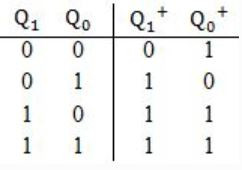The counter is built as a synchronous sequential circuit using T flip-flops. The expressions for T1 and T0 are

 ABCD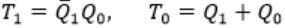Digital-Logic-Design       Sequential-Circuits       GATE 2017 [Set-2]       Video-Explanation
Question 21 Explanation:By using above excitation table,Question 22

Consider the Boolean operator with the following properties:Then x#y is equivalent to

 AB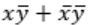C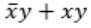DDigital-Logic-Design       Boolean-Algebra       GATE 2016 [Set-1]       Video-Explanation
Question 22 Explanation: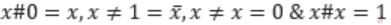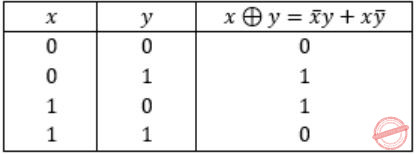Ex-OR satisfies all the properties. Hence,Question 23

The 16-bit 2’s complement representation of an integer is 1111 1111 1111 0101; its decimal representation is __________.

 A -11 B -12 C -13 D -14
Digital-Logic-Design       Number-Systems       GATE 2016 [Set-1]       Video-Explanation
Question 23 Explanation:
Given number is 1111 1111 1111 0101.
It is a negative number because MSB is 1.
Magnitude of 1111 1111 1111 0101 is 2’s complement of 1111 1111 1111 0101.
1111 1111 1111 0101
0000 0000 0000 1010 : 1’s Complement
0000 0000 0000 1011 : 2’s complement
= (11)10
Hence, 1111 1111 1111 0101 = -11
 Question 24

We want to design a synchronous counter that counts the sequence 0-1-0-2-0-3 and then repeats. The minimum number of J-K ﬂip-ﬂops required to implement this counter is __________.

 A 4 B 5 C 6 D 7
Digital-Logic-Design       Sequential-Circuits       GATE 2016 [Set-1]       Video-Explanation
Question 24 Explanation:
Given sequence is 0-1-0-2-0-3
There are 3 transitions from 0.
Hence ⌈log23⌉ = 2 bits have to be added to the existing 2 bits to represent 4 unique states.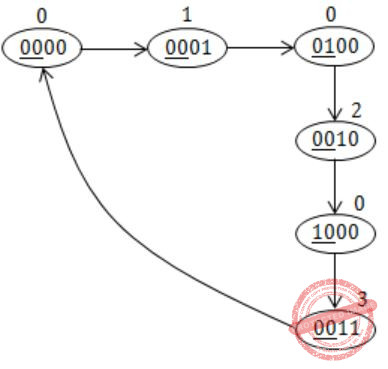Question 25

Consider the two cascaded 2-to-1 multiplexers as shown in the ﬁgure.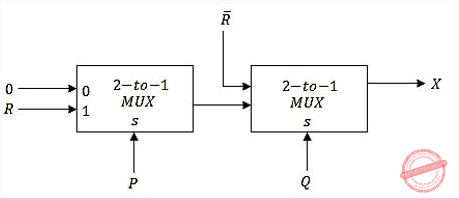The minimal sum of products form of the output X is

 ABC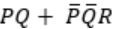DDigital-Logic-Design       Multiplexer       GATE 2016 [Set-1]       Video-Explanation
Question 25 Explanation:
Output of 1st MUX isNowQuestion 26

Consider a carry lookahead adder for adding two n-bit integers, built using gates of fan-in at most two. The time to perform addition using this adder is __________.

 A Θ(1) B Θ(log(n)) C Θ(√n) D Θ(n)
Digital-Logic-Design       Carry-Look-Ahead-Buffer       GATE 2016 [Set-1]       Video-Explanation
Question 26 Explanation:
Formula: θ(logk (n))
Where n is number of bits added
and k is fan-in of the gates.
As we are adding n-bit numbers and fan-in is at most 2,
the solution is θ(log2 (n)).
 Question 27

Consider an eight-bit ripple-carry adder for computing the sum of A and B, where A and B are integers represented in 2’s complement form. If the decimal value of A is one, the decimal value of B that leads to the longest latency for the sum to stabilize is _________.

 A -1 B -2 C -3 D -4
Digital-Logic-Design       Adder       GATE 2016 [Set-2]       Video-Explanation
Question 27 Explanation:
In the question, longest LATENCY means longest DELAY for the sum to get settle.
If we do 2’s complement of 1 = 0000 0001, we get -1 = “1111 1111”So, if B = -1, every carry bit is 1.
 Question 28

Let, x1⊕x2⊕x3⊕x4 = 0 where x1, x2, x3, x4 are Boolean variables, and ⊕ is the XOR operator. Which one of the following must always be TRUE?

 A x1x2x3x4 = 0 B x1x3+x2 = 0 CD x1 + x2 + x3 + x4 = 0
Digital-Logic-Design       Boolean-Algebra       GATE 2016 [Set-2]       Video-Explanation
Question 28 Explanation:
Given expression is,
x1 ⊕ x2 ⊕ x3 ⊕ x4 = 0 —–(1)
A) x1x2x3 x4 = 0
Put x1 = 1, x2 = 1, x3 = 1, x4 = 1
The given equation will be zero, i.e.,
1 ⊕ 1 ⊕ 1 ⊕ 1 = 0
But,
x1x2x3 x4 ≠ 0
So, false.
B) x1x3 + x2 = 0
Put x1 = 1, x2 = 1, x3 = 0 , x4 = 0
The given equation will be zero, i.e.,
1 ⊕ 1 ⊕ 0 ⊕ 0 = 0
But,
x1x3 + x2 ≠ 0
So, false.
D) x1 + x2 + x3 + x4 = 0
Let x1=1, x2=1, x3=0, x4=0
The given equation will be zero, i.e.,
1 ⊕ 1 ⊕ 0 ⊕ 0 = 0
But,
x1 + x2 + x3 + x4 ≠ 0
So, false.
(i) True.
 Question 29

Let X be the number of distinct 16-bit integers in 2’s complement representation. Let Y be the number of distinct 16-bit integers in sign magnitude representation.

Then X-Y is _________.

 A 1 B 2 C 3 D 4
Digital-Logic-Design       Number-Systems       GATE 2016 [Set-2]       Video-Explanation
Question 29 Explanation:
X = 216
Since range is – 215 to 215 – 1
Y = 216 – 1
Here, +0 and -0 are represented separately.
X – Y = 216 – (216 – 1)
= 1
 Question 30

Consider a 4-bit Johnson counter with an initial value of 0000. The counting sequence of this counter is

 A 0, 1, 3, 7, 15, 14, 12, 8, 0 B 0, 1, 3, 5, 7, 9, 11, 13, 15, 0 C 0, 2, 4, 6, 8, 10, 12, 14, 0 D 0, 8, 12, 14, 15, 7, 3, 1, 0
Digital-Logic-Design       Sequential-Circuits       GATE 2015 [Set-1]
Question 30 Explanation:
In a Johnson’s counter LSB is complemented and a circular right shift operation has to be done to get the next state.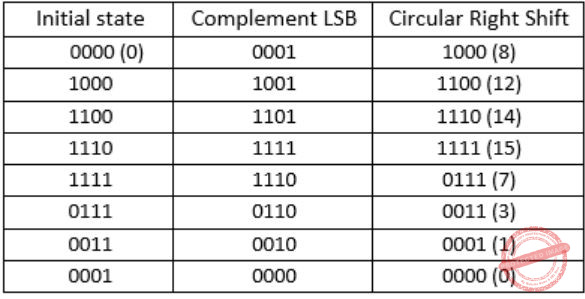The state sequence is 0,8,12,14,15,7,3,1,0.
 Question 31

The binary operator ≠ is defined by the following truth table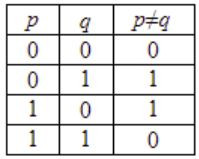Which one of the following is true about the binary operator ≠?

 A Both commutative and associative B Commutative but not associative C Not commutative but associative D Neither commutative nor associative
Digital-Logic-Design       Truth Table and Boolean Expressions       GATE 2015 [Set-1]
Question 31 Explanation:
It is clear that from the truth table, the binary operation # is equivalent to XOR i.e., ⊕, which satisfies both commutative and associative i.e., p#q q#p and p#(q#r) (p#q)#r.
 Question 32

A positive edge-triggered D flip-flop is connected to a positive edge-triggered JK flipflop as follows. The Q output of the D flip-flop is connected to both the J and K inputs of the JK flip-flop, while the Q output of the JK flip-flop is connected to the input of the D flip-flop. Initially, the output of the D flip-flop is set to logic one and the output of the JK flip-flop is cleared. Which one of the following is the bit sequence (including the initial state) generated at the Q output of the JK flip-flop when the flip-flops are connected to a free-running common clock? Assume that J = K = 1 is the toggle mode and J = K = 0 is the state-holding mode of the JK flip-flop. Both the flip-flops have non-zero propagation delays.

 A 0110110… B 0100100… C 011101110… D 011001100…
Digital-Logic-Design       Sequential-Circuits       GATE 2015 [Set-1]
Question 32 Explanation:
The circuit for the given data isThe characteristic equations are
QDN=D=QJK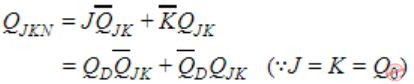The state table and state transition diagram are as follows: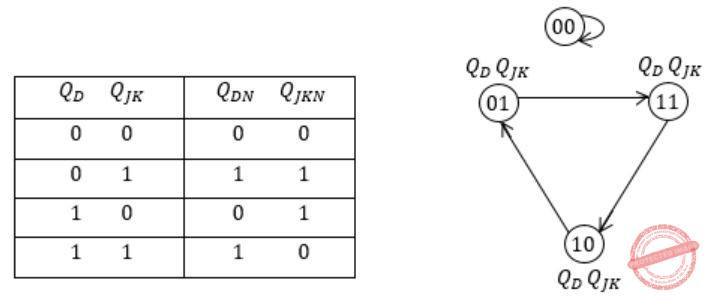Consider QDQJK=10 as initial state because in the options QJK=0 is the initial state of JK flip-flop.
The state sequence is0 → 1 → 1 → 0 → 1 → 1
∴ Option (a) is the answer.
 Question 33

The minimum number of JK flip-flops required to construct a synchronous counter with the count sequence (0, 0, 1, 1, 2, 2, 3, 3, 0, 0,……) is ___________.

 A 2 B 3 C 4 D 5
Digital-Logic-Design       Sequential-Circuits       GATE 2015 [Set-2]
Question 33 Explanation:
Count sequence mentioned is
00
00
01
01
10
10
11
11
In the above sequence two flip-flop’s will not be sufficient. Since we are confronted with repeated sequence, we may add another bit to the above sequence.
000
100
001
101
010
110
011
111
Now and every count is unique, occurring only once.
So finally 3-flip flops is required.
 Question 34

The number of min-terms after minimizing the following Boolean expression is ______.

`      [D′ + AB′ + A′C + AC′D + A′C′D]′    `
 A 1 B 2 C 3 D 4
Digital-Logic-Design       Boolean-Algebra       GATE 2015 [Set-2]
Question 34 Explanation:
Lets simplify it
[D’ + AB’ + A’C + AC’D + A’C’D]’
[D’ + AB’ + A’C + C’D (A + A’)’]’ (since A+A’ = 1)
[AB’ + A’C + (D’ + C’) (D’ + D)]’ (since D’ + D =1)
[AB’ + A’C + D’ + C’]’
[AB’ + (A’ + C’) (C + C’) + D’]’
[AB’ + A’ + C’ + D’]’
[(A + A’) (A’ + B’) + C’ + D’]’
[A’ + B’ + C’ + D’]’
Apply de-morgan’s law,
ABCD
 Question 35

A half adder is implemented with XOR and AND gates. A full adder is implemented with two half adders and one OR gate. The propagation delay of an XOR gate is twice that of an AND/OR gate. The propagation delay of an AND/OR gate is 1.2 microseconds. A 4-bit ripple-carry binary adder is implemented by using four full adders. The total propagation time of this 4-bit binary adder in microseconds is ____________.

 A 19.1 B 19.2 C 18.1 D 18.2
Question 35 Explanation:Here, each Full Adder is taking 4.8 microseconds. Given adder is a 4 Bit Ripple Carry Adder. So it takes 4*4.8 = 19.2 microseconds.
 Question 36

The total number of prime implicants of the function f(w,x,y,z) = Σ(0, 2, 4, 5, 6, 10) is ______.

 A 3 B 4 C 2 D 1
Digital-Logic-Design       K-Map       GATE 2015 [Set-3]
Question 36 Explanation:Total 3 prime implicants are there.
 Question 37

Consider the following Boolean expression for F:

`   F(P, Q, R, S) = PQ + P'QR + P'QR'S`

The minimal sum-of-products form of F is

 ABC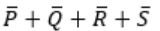DDigital-Logic-Design       Boolean-Algebra       GATE 2014 [Set-1]
Question 37 Explanation:
PQ + P’QR + P’QR’S
= Q(P+P’R) + P’QR’S
= Q(P+R) + P’QR’S
= QP + QR + P’QR’S
= QP + Q(R + P’R’S)
= QP + Q( R + P’S)
= QP + QR + QP’S
= Q(P+P’S) + QR
= Q(P+S)+ QR
= QP + QS + QR
 Question 38

The base (or radix) of the number system such that the following equation holds is_________.

`        312/20 = 13.1     `
 A 5 B 6 C 7 D 8
Digital-Logic-Design       Number-Systems       GATE 2014 [Set-1]
Question 38 Explanation:
Let base of the number system is r.
(3r2 + r + 2) / 2r= (r+3+1/r)
(3r2 + r + 2) / 2r= (r2+3r+1) / r
(3r2 + r + 2) = (2r2+6r+2)
r2 -5r = 0
Therefor r = 5
 Question 39

Consider a 4-to-1 multiplexer with two select lines S1 and S0, given belowThe minimal sum-of-products form of the Boolean expression for the output F of the multiplexer is

 ABCD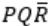Digital-Logic-Design       Multiplexer       GATE 2014 [Set-1]
Question 39 Explanation:
F(P,Q,R) = P’Q’(0) + P’Q (1) + PQ’(R) + PQ(R’)
= P’Q + PQ’R + PQR’
= Q(P’ + P R’) + PQ’R
= Q(P’ + R’) + PQ’R
= P’Q + QR’ + PQ’R
 Question 40

The dual of a Boolean function F(x1, x2, …, xn, +, ⋅, ‘), written as FD, is the same expression as that of F with + and ⋅ swapped. F is said to be self-dual if F = FD. The number of self-dual functions with n Boolean variables is

 A 2n B 2(n-1) C 2(2n ) D 2(2(n-1) )
Digital-Logic-Design       Self-Dual-Function       GATE 2014 [Set-2]
Question 40 Explanation:
Number of possible minterms = 2n.
Number of mutually exclusive pairs of minterms = 2n-1.
There are 2 choices for each pair i.e., we can choose one of the two minterms from each pair of minterms for the function.
Therefore number of functions = 2 x 2 x …. 2n-1 times.
= 2(2(n-1))
 Question 41

Let k = 2n. A circuit is built by giving the output of an n-bit binary counter as input to an n-to-2n bit decoder. This circuit is equivalent to a

 A k-bit binary up counter. B k-bit binary down counter. C k-bit ring counter. D k-bit Johnson counter.
Digital-Logic-Design       Sequential-Circuits       GATE 2014 [Set-2]
Question 41 Explanation:
A ring counter is a circular shift register with only one flip-flop being set at any particular time and all others are cleared.
A n x 2n decoder is a combinational circuit with only one output line has one and all others (2n-1) have zeros.
A n-bit binary Counter produces outputs from 0 to 2n i.e 000…00 to 111…11 and repeats.
The n x 2n Decoder gets the input (000..00 to 111…11 ) from the binary counter and only one output line has one and rest have zeros.
This circuit is equivalent to a 2n – bit ring counter.
 Question 42

Consider the equation (123)5 = (x8)y with x and y as unknown. The number of possible solutions is __________.

 A 3 B 5 C 6 D 7
Digital-Logic-Design       Number-Systems       GATE 2014 [Set-2]
Question 42 Explanation:
First we have to fullfill all the conditios,
(123)5 = (x8)y
In R.H.S. since y is base so y should be greater than x and 8, i.e.,
y > x
y > 8
Now, to solve let’s change all the above bases number into base 10 number,
52 × 1 +2 × 5 + 3 = y × x + 8
38 = xy + 8
xy = 30
⇒ yx = 30
So the possible combinations are
(1,30), (2,15), (3,10), (5,6)
But we will reject (5,6) because it violates the condition (y > 8).
So, total solutions possible is 3.
 Question 43

Consider the following minterm expression for F:

`  F(P,Q,R,S) = Σ0,2,5,7,8,10,13,15  `

The minterms 2, 7, 8 and 13 are ‘do not care’ terms. The minimal sum-of-products form for F is:

 A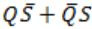BC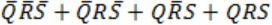DDigital-Logic-Design       Number-Systems       GATE 2014 [Set-3]
Question 43 Explanation:Question 44

Consider the following combinational function block involving four Boolean variables x, y, a, b where x, a, b are inputs and y is the output.

```f (x, y, a, b)
{
if (x is 1) y = a;
else y = b;
}
```

Which one of the following digital logic blocks is the most suitable for implementing this function?

 A Full adder B Priority encoder C Multiplexor D Flip-flop
Digital-Logic-Design       Boolean-Variables       GATE 2014 [Set-3]
Question 44 Explanation:
A 2×1 Multiplexer is most suitable for implementing the function.
x is the select line, I0 is ‘b’ and I1 is a.
The output line, y = xa + x’b
 Question 45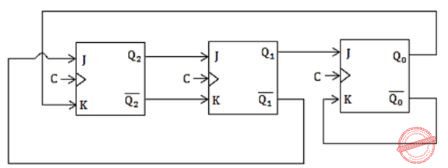The above synchronous sequential circuit built using JK flip-flops is initialized with Q2Q1Q0 = 000. The state sequence for this circuit for the next 3 clock cycles is

 A 001, 010, 011 B 111, 110, 101 C 100, 110, 111 D 100, 011, 001
Digital-Logic-Design       Flip-Flops       GATE 2014 [Set-3]
Question 45 Explanation:Question 46

Let ⊕ denote the Exclusive OR (XOR) operation. Let ‘1’ and ‘0’ denote the binary constants. Consider the following Boolean expression for F over two variables P and Q:

`     F(P,Q) = ((1⊕P)⊕(P⊕Q))⊕((P⊕Q)⊕(Q⊕0)) `

The equivalent expression for F is

 A P+Q B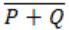C P⨁Q DDigital-Logic-Design       Number-Systems       GATE 2014 [Set-3]
Question 46 Explanation:
((1 ⊕ P) ⊕ (P ⊕ Q)) ⊕ ((P ⊕ Q) ⊕ (Q ⊕ 0))
⊕ is associative i.e P ⊕ (Q ⊕ R) = (P⊕Q) ⊕ R.
P ⊕ P = 0, 1 ⊕ P = P’ and 0 ⊕ Q = Q
(1 ⊕ P) ⊕ ((P ⊕ Q) ⊕ (P ⊕ Q)) ⊕ (Q ⊕ 0)
= P’⊕ (0) ⊕ Q
= P’ ⊕ Q
= (P ⊕ Q)’
 Question 47

The smallest integer that can be represented by an 8-bit number in 2’s complement form is

 A -256 B -128 C -127 D 0
Digital-Logic-Design       Number-Systems       GATE 2013
Question 47 Explanation:
The range of 8-bit signed numbers representable is – 2n-1 to 2n-1 -1.
The smallest 8-bit 2’s complement number is 1000 0000.
MSB is 1. So it is a negative number.
To know the magnitude again take 2’s complement of 1000 0000.
1000 0000
0111 1111 ← 1’s complement
1000 0000 ← 2’s complement (1’s complement +1)
= 128
-128 is 1000 0000 in 2’s complement representation.
 Question 48

In the following truth table, V = 1 if and only if the input is valid.What function does the truth table represent?

 A Priority encoder B Decoder C Multiplexer D Demultiplexer
Digital-Logic-Design       Combinational-Circuits       GATE 2013
Question 48 Explanation:
It is a 22 × 2 encoder. The inputs have priorities. So, it is a priority encoder.
 Question 49

Which one of the following expressions does NOT represent exclusive NOR of x and y?

 A xy+x’y’ B x⊕y’ C x’⊕y D x’⊕y’
Digital-Logic-Design       Number-Systems       GATE 2013
Question 49 Explanation:
x ⊕ y = x’y + xy’
x’ ⊕ y’ = xy’ + x’y = x⊕y. Hence option D is correct.
 Question 50

The truth tablerepresents the Boolean function

 A X B X + Y C X ⊕ Y D Y
Digital-Logic-Design       Boolean-Algebra       GATE 2012
Question 50 Explanation:
f(X,Y) = XY’ + XY = X(Y’ + Y) = X
There are 50 questions to complete.

Register Now